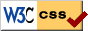# Notes on Diffy Qs - SAGE demonstrations for section 3.5

Press the Activate buttons below to launch the SAGE demonstration. After the sage cell is evaluated you should be able to interact with it to change numbers with sliders. You may have to wait a little before the graph appears. Be patient.

## Two dimensional linear system

Plot the vector field and a solution to

$\left[ \begin{matrix} x' \\ y' \end{matrix} \right] = \left[ \begin{matrix} a & b \\ c & d \end{matrix} \right] \left[ \begin{matrix} x \\ y \end{matrix} \right]$

The eigenvalues are computed and printed, the vector field is drawn, and a solution for $0 < t < 10$ is drawn. You can change the matrix and the initial value of the solution.

## Two dimensional linear system with given eigenvalues

Plot the vector field and a solution to

$\left[ \begin{matrix} x' \\ y' \end{matrix} \right] = P \left[ \begin{matrix} x \\ y \end{matrix} \right]$

where $P$ is some matrix with eigevalues $a \pm bi$, or $a$ and $c$ (set $b=0$ to get the two real eigenvalues). The vector field is drawn, and a solution for $0 < t < 10$ is drawn.

The code is mainly due to Ryan Burkhart, it was then slightly modified by Jiří Lebl.